# Create a Standard Loan

A standard loan in Fairways Debt is a debt transaction subscribed by an entity with one counterparty (internal or external, the Lender) at a given interest rate.

Its details include: the principal amount, the interest rate, the repayment date, etc.

The term standard loan in Fairways Debt refers to all loans that are not characterized by more specific features (like the Ongoing Loans or the PIK Loans).

Standard loans include one initial drawing, equal to the loan principal, an interest payment schedule and an amortization schedule. The related periods, payment dates, interest calculations and amortization modes can be very simple or very complicated.

Navigate to the Debt & Derivatives Application
2. Select a customer account.
3. Navigate to Applications > Debt & Derivatives.

Create a Standard Loan
1. Click Add Transaction > Loan > Standard Loan.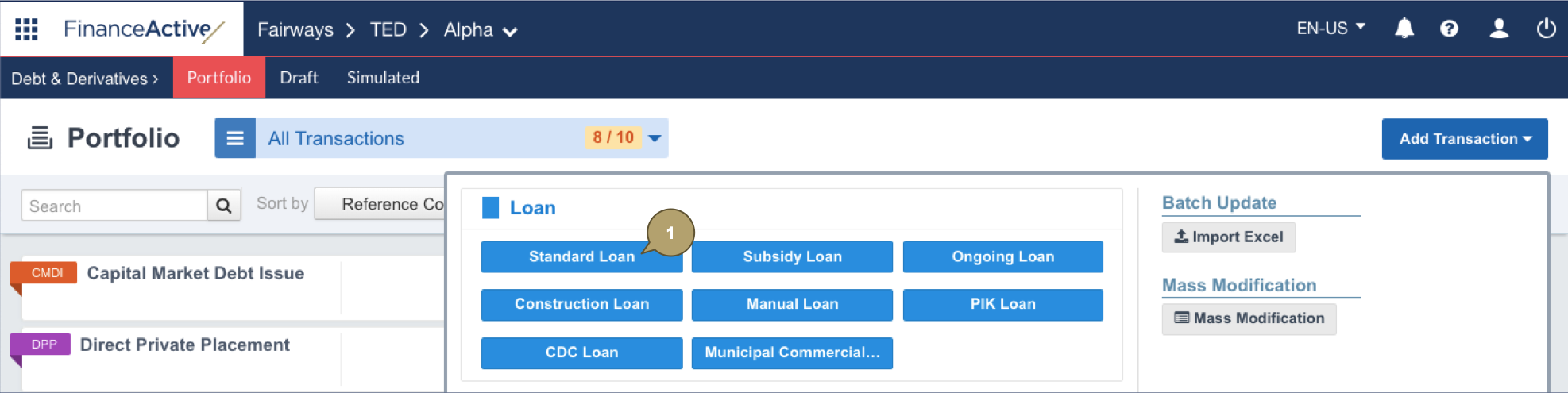1. Complete the form with all relevant details.

Note: Fields marked with an asterisk * are mandatory.

Field

Description

Reference Code

Unique reference of the transaction. Identifies the transaction in the portfolio.

Note: The reference must be unique among all the entities managed in the account.

External Reference Code

Used by external systems to identify the transaction. Used when transactions are imported from or exported to another system.

Principal

Principal amount of the loan.

Currency

Currency of the principal amount.

Borrower

Borrowing entity in the system. Only entities in the current organization can be managed by the current user.

Lender

Can be either internal (an entity in the system) or external (a commercial bank).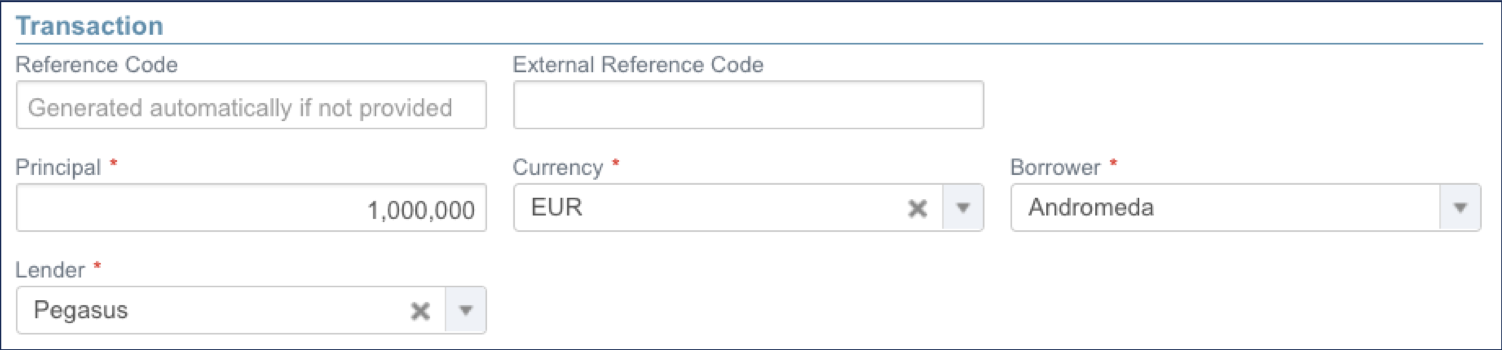Field

Description

Date at which the transaction has been traded. From that date, the system takes the transaction into account as an item of the portfolio.

Start Date

Unadjusted start date of the transaction.

Note: The start date must be later or equal to the trade date.

End Date

Unadjusted end date of the transaction.

Note: The end date must be later than the start date.

First Roll Date

Unadjusted date of the first payment (useful when the first period is a long or short stub).

Note: The first roll date must be later than the start date and earlier than the end date.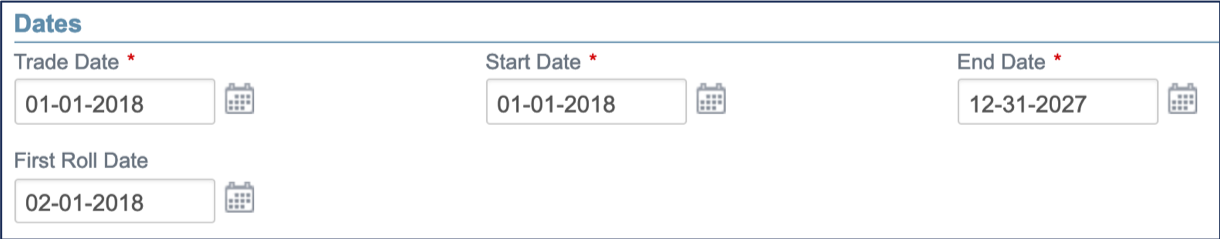Field

Description

Availability Period

Indicates if the loan includes drawings and amortization:

• No: The full principal is drawn at the start of the transaction.
• Yes, with amortization: The principal is drawn over a period of time (multiple drawdown dates) and the principal is amortizing.
• Yes, without amortization: The principal is drawn over a period of time (multiple drawdown dates).

Notes:

• You cannot edit this field once you created the loan.
• Additional fields display depending on the selection.

End of Availability Period

Note: This field displays if the availability period is enabled.

Indicates the last date at which drawings can be made.

Note: If the availability period is enabled with no amortization, the end of availability period must be earlier than the end date.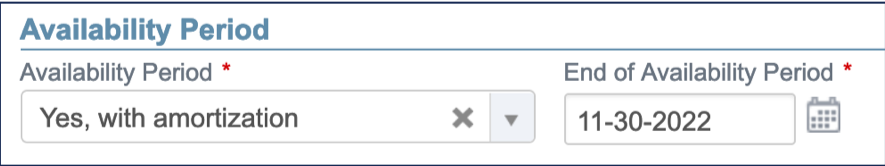Field

Description

Amortization Type

Amortization mode.

Consolidation Start Date

Note: This field is only available for drawings with amortization.

Indicates the date at which amortization can start.

Extended Constant Annuity Date

Note: This field displays if extended constant annuities are selected as amortization type.

Indicates the date until which constant annuities will be computed (a transaction with a maturity date corresponding to the extended constant annuity date would amortize to zero at this date).Field

Description

Index

Name of the index used to calculate the rate value, e.g. FIXED for a fixed rate.

Note: The field beside displays depending on the index selected.

Only a fixed index can be defined if constant annuities or extended constant annuities are selected as the amortization type.

Interest Rate

Fixed rate value in percentage.

Note: This field displays depending on the index selected.

Initial Interest Rate

Initial rate of the custom index.

Note: This field displays depending on the index selected.

Spread (or margin) value in percentage.

Note: This field displays depending on the index selected.

Day Count Convention

Used to compute the day fraction of an interest accrual period.

Capitalized Interest

Defines whether the interest amount should be paid at the payment date, or added to the principal (and included into the outstanding balance for the following periods).

Defines whether the interest amount should be paid at the start of the period.

Equivalent Compounding Rate

Note: This field displays if a fixed index is selected.

Enables the computation of the equivalent compounding rate.

This rate is equivalent to the interest rate but paid with a different frequency, with compound interests.

The effective rate is then the yearly rate which corresponds to the interest rate divided by the selected frequency, and compound at the selected frequency. As an example, for an interest rate of 2% and a bi-annual capitalization frequency (ie. twice a year), the effective rate is the rate equivalent to compounding at a 1% interest rate over 2 periods of 6 months, which is 2.2121%.

Furthermore, a period equivalent rate is computed in relation to the payment frequency of the transaction. This equivalent rate is equivalent to the effective rate if compound at the payment frequency. Each payment is computed with this equivalent rate multiplied by the payment frequency and using the day count convention. For example, if the principal is 1,000,000 CAD, the day count convention is Actual/360 and the equivalent rate is 0.20 % for 12 payments per year (monthly payments), then the payment for a period of 28 days would be: 1M x (12 x 0.20%) x 28/360 = 1866.67 CAD.

Annual Capitalization Frequency

Note: This field displays if the equivalent compounding rate is selected.

Number of capitalizations in the year.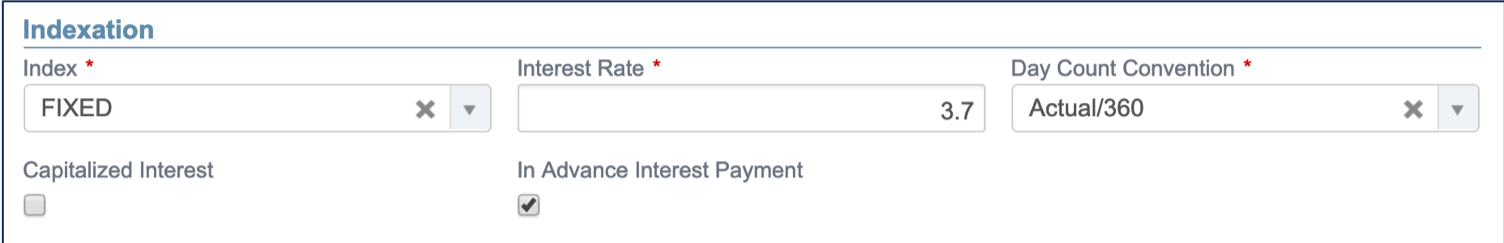Field

Description

Frequency

Frequency of the payments.

Note: The available values correspond to a fraction of the annual capitalization frequency, if selected.

Adjustment mode for the interest calculation. The nominal start and end dates of the accrual period will be adjusted accordingly before computing the interest amount.

Adjustment mode for the payment date.

Last Stub Period

Defines whether the last period should be a short or long stub when it does not match the selected frequency:

• Short Stub: The payment date just before the end date should be respected.
• Long Stub: The payment date just before the end date is replaced with the payment occurring at the end date.

Note: If neither stub is selected, the short stub applies by default.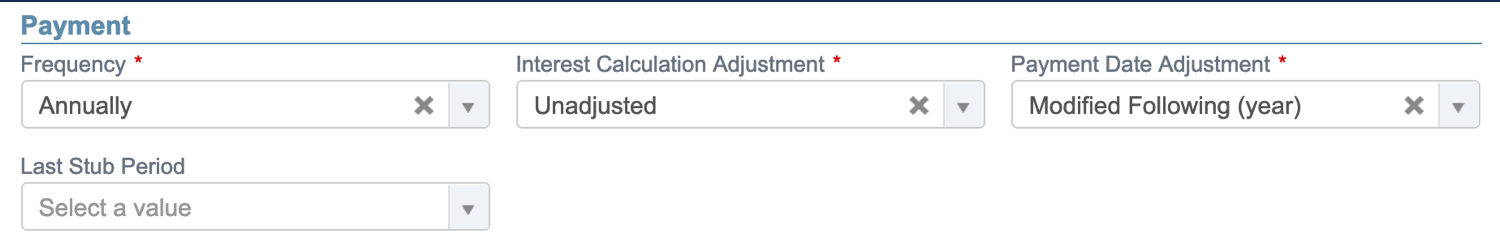Enter the required custom attributes, if any.

1. Enable Show Details after Validation to automatically open the new standard loan profile once created.
2. Click Apply to create the standard loan.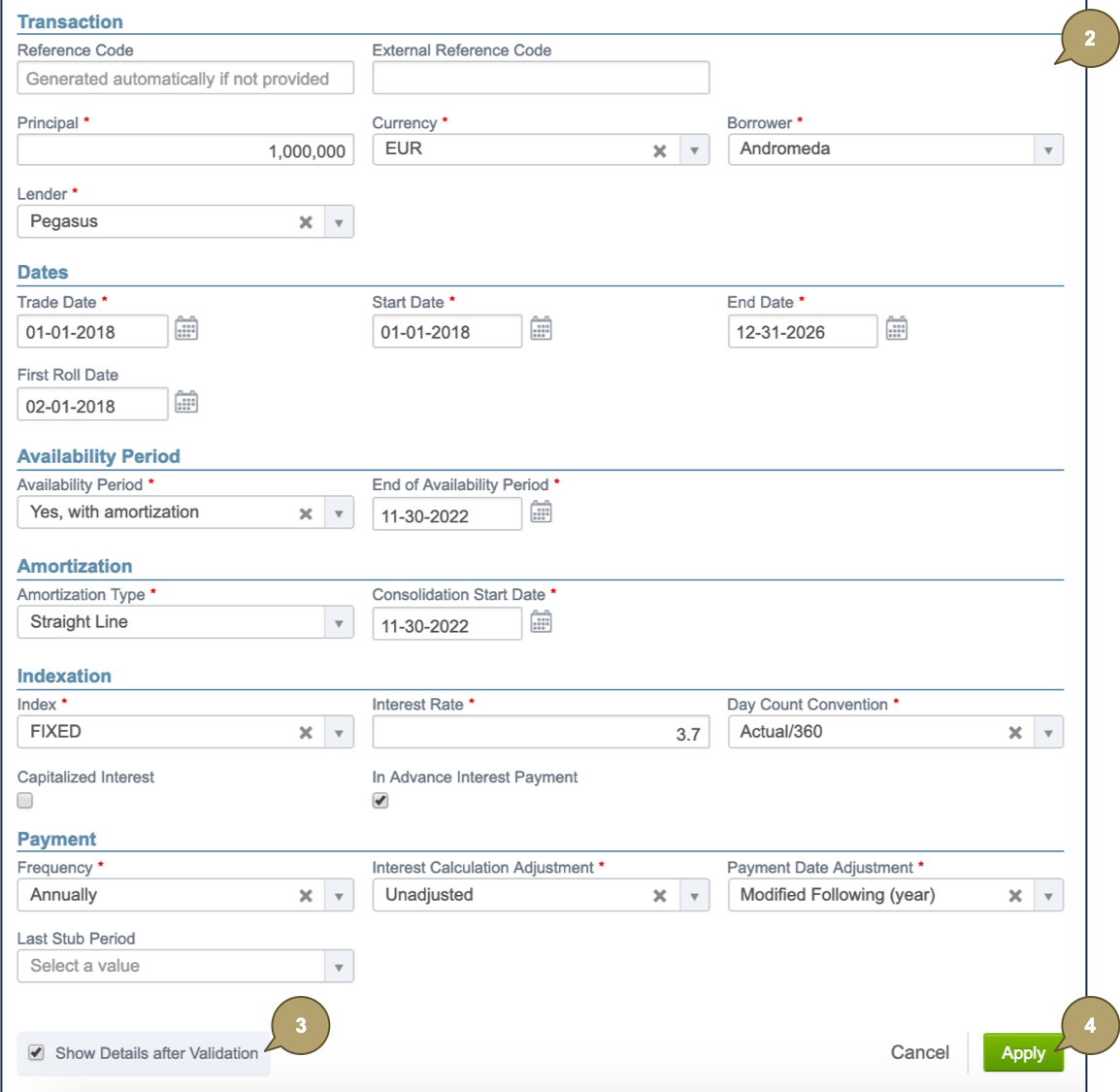The new standard loan displays in the draft portfolio.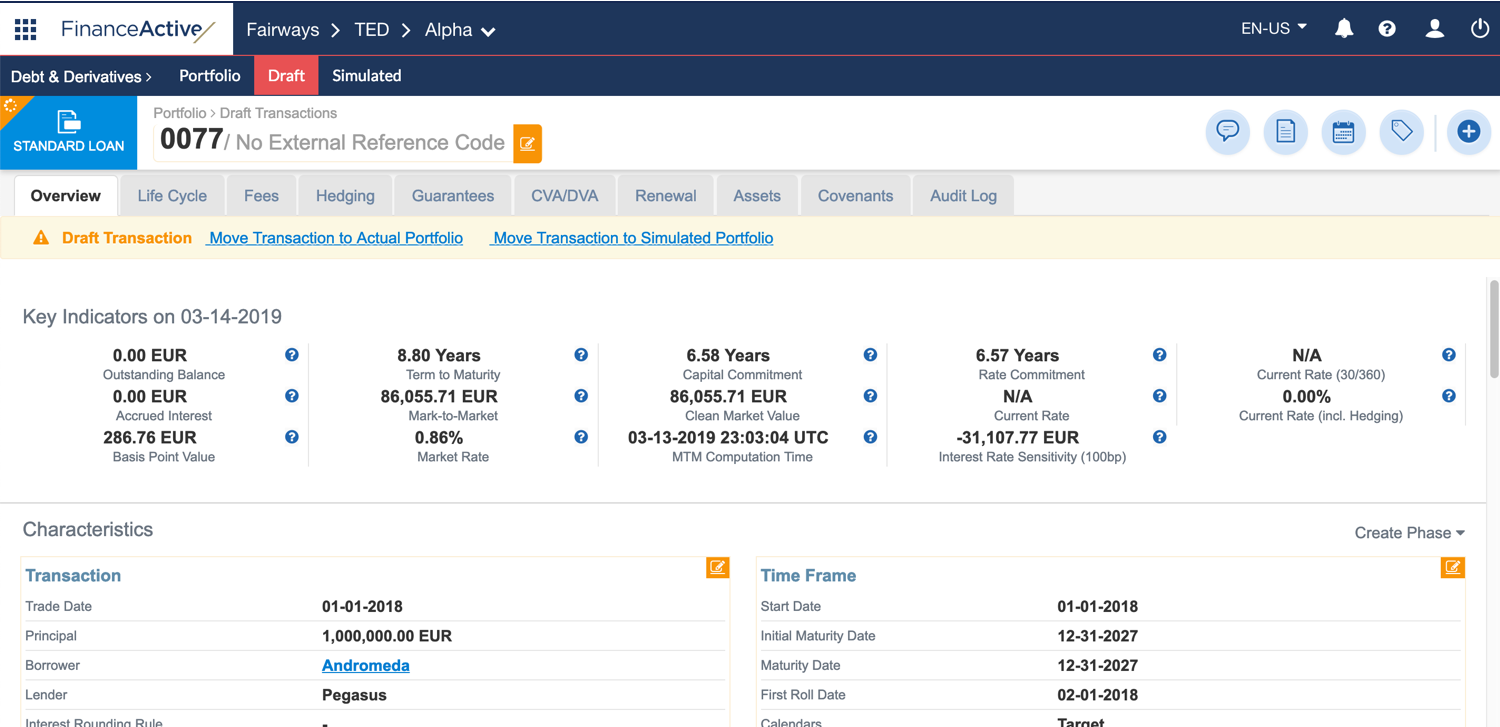What's Next?